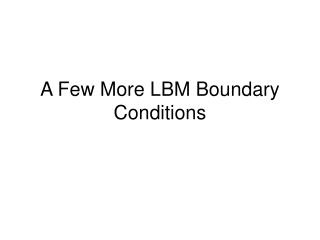# A Few More LBM Boundary Conditions - PowerPoint PPT PresentationDownload PresentationA Few More LBM Boundary Conditions

A Few More LBM Boundary ConditionsDownload Presentation## A Few More LBM Boundary Conditions

- - - - - - - - - - - - - - - - - - - - - - - - - - - E N D - - - - - - - - - - - - - - - - - - - - - - - - - - -
##### Presentation Transcript

1. A Few More LBM Boundary Conditions

2. Key paper: • Zou, Q. and X. He, 1997, On pressure and velocity boundary conditions for the lattice Boltzmann BGK model, Phys. Fluids 9, 1591-1598.

3. Choices • Specify density (i.e., pressure via EOS) • Velocity computed • Dirichlet • Specify velocity • Density/pressure computed • Von Neumann • Lots of temporal/spatial flexibility

4. D2Q9 BCs • For example: • f(4,7,8) = function of f(1,2,3,5,6) and BC type Out In

5. Velocity/Flux BCs • Need to solve for r, f4, f7, f8 • Need 4 equations • The macroscopic density formula is one equation:

6. Velocity/Flux BCs • The macroscopic velocity formula gives two equations: • x-direction: • y-direction: Components of ea are all unit vectors Assuming ux = 0

7. Velocity/Flux BCs • Finally, we assume bounceback of f perpendicular to boundary for a fourth equation:

8. Velocity/Flux BCs • Two equations have the directional density unknowns f4, f7 and f8 in common, so rewrite them with those variables on the left hand side:

9. Velocity/Flux BCs • Equating them gives: • Solving for r:

10. Velocity/Flux BCs • Solving the bounceback equation for f4: • In detail, part of this is:

11. Velocity/Flux BCs • Solving …:

12. Velocity/Flux BCs • Solving …:

13. Velocity/Flux BCs • // Zou and He velocity BCs on north side. • for( i=0; i<ni; i++) • { • fi = ftemp[nj-1][i]; • rho0 = ( fi + fi + fi • + 2.*( fi + fi + fi)) / ( 1. + uy0); • ru = rho0*uy0; • fi = fi - (2./3.)*ru; • fi = fi - (1./6.)*ru + (1./2.)*( fi-fi); • fi = fi - (1./6.)*ru + (1./2.)*( fi-fi); • }

14. Pressure/Density Boundaries • Dirichlet boundary conditions constrain the pressure/density at the boundaries • Solution is closely related to that for velocity boundaries • A density r0 is specified and velocity is computed • Specifying density is equivalent to specifying pressure since there is an equation of state (EOS) relating them directly • For single component D2Q9 model, the relationship is simply P = RTr with RT = 1/3. • More complex EOS applies to single component multiphase models • We assume that velocity tangent to the boundary is zero and solve for the component of velocity normal to the boundary.

15. Pressure/Density Boundaries • Assume that velocity tangent to the boundary is zero and solve for the component of velocity normal to the boundary • Need to solve for v, f4, f7 and f8

16. Pressure/Density Boundaries

17. Pressure/Density Boundaries

18. Pressure/Density Boundaries • // Zou and He pressure boundary on north side. • for( i=0; i<ni; i++) • { • fi = ftemp[nj-1][i]; • uy0 = -1. + ( fi + fi + fi • + 2.*( fi + fi + fi)) / rho0; • ru = rho0*uy0; • fi = fi - (2./3.)*ru; • fi = fi - (1./6.)*ru + (1./2.)*( fi-fi); • fi = fi - (1./6.)*ru + (1./2.)*( fi-fi); • }

19. Exercise • Create a new version of your code that includes constant pressure boundaries at x = 0 and x = Lx. • Plot the observations and expected Poiseuille velocity profile on the same graph